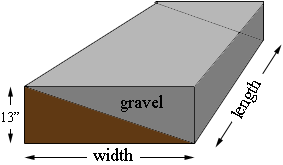SEARCH HOMEMath Central Quandaries & QueriesQuestion from Robert, a parent: I have an area that is 55 feet long x 75 feet wide and goes from 0 to 13 inches deep. How much gravel do I need to make it level?Hi Robert,

Can you give me some more information? Is one side 13 inches deep and the opposite side 0 inches deep? If so, which side, the 55 foot side or the 75 foot side?

Penny

Robert wrote back

Ms.Penny,
the north section is 0 inches (at grade) the south section is 13 inches lower and the and the area is rectangle shape, 55' feet x 75' feet.

What you have is a large rectangular box. The length and width are 55 feet and 75 feet and the height 13 inches. I don't know which dimension is the length and which is the width but it doesn't matter. You slice the box in half along along a diagonal plane and above the plane is the gravel. Here is my diagram (not to scale).Thus the volume you need is half the volume of the box.

Use our volume calculator to find the volume of the box in cubic yards and then divide this volume by 2.

I hope this helps,
PennyMath Central is supported by the University of Regina and The Pacific Institute for the Mathematical Sciences.